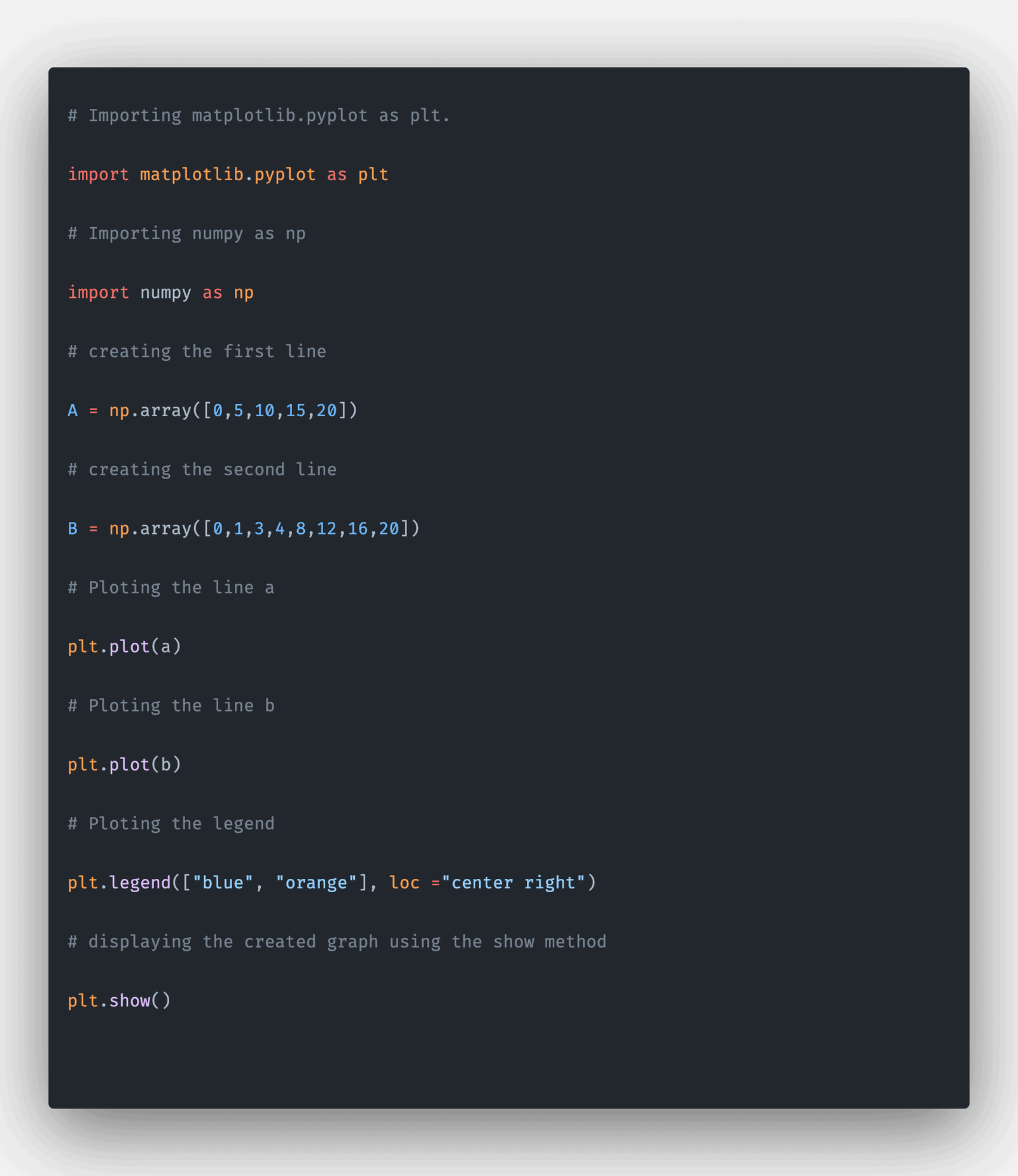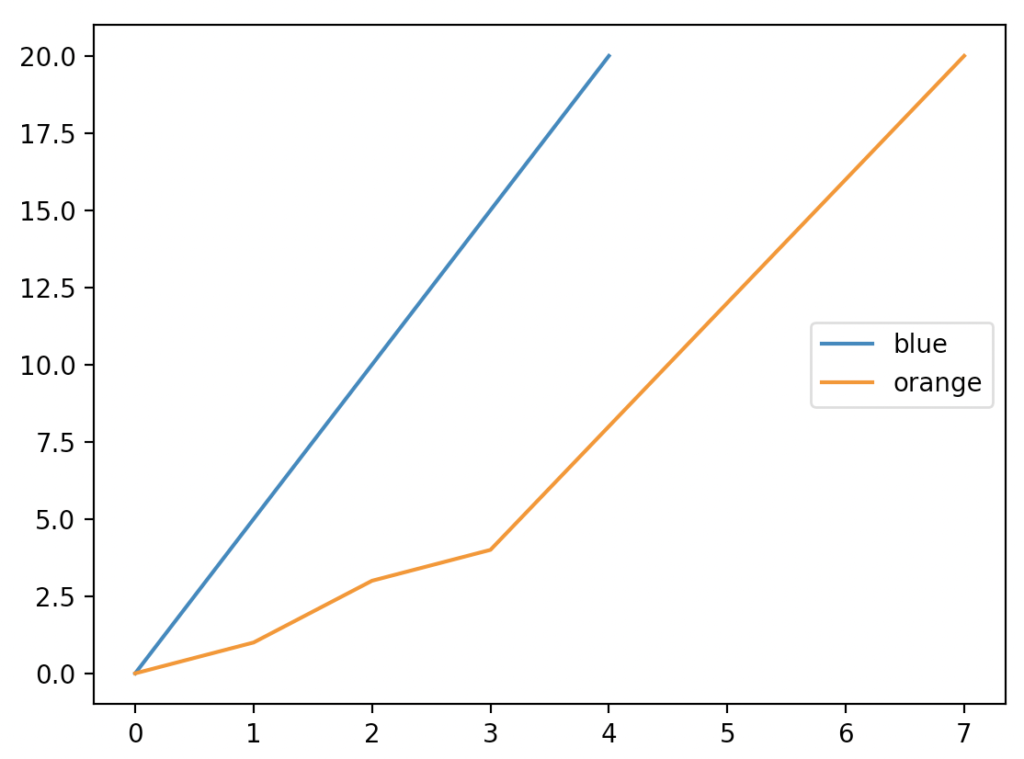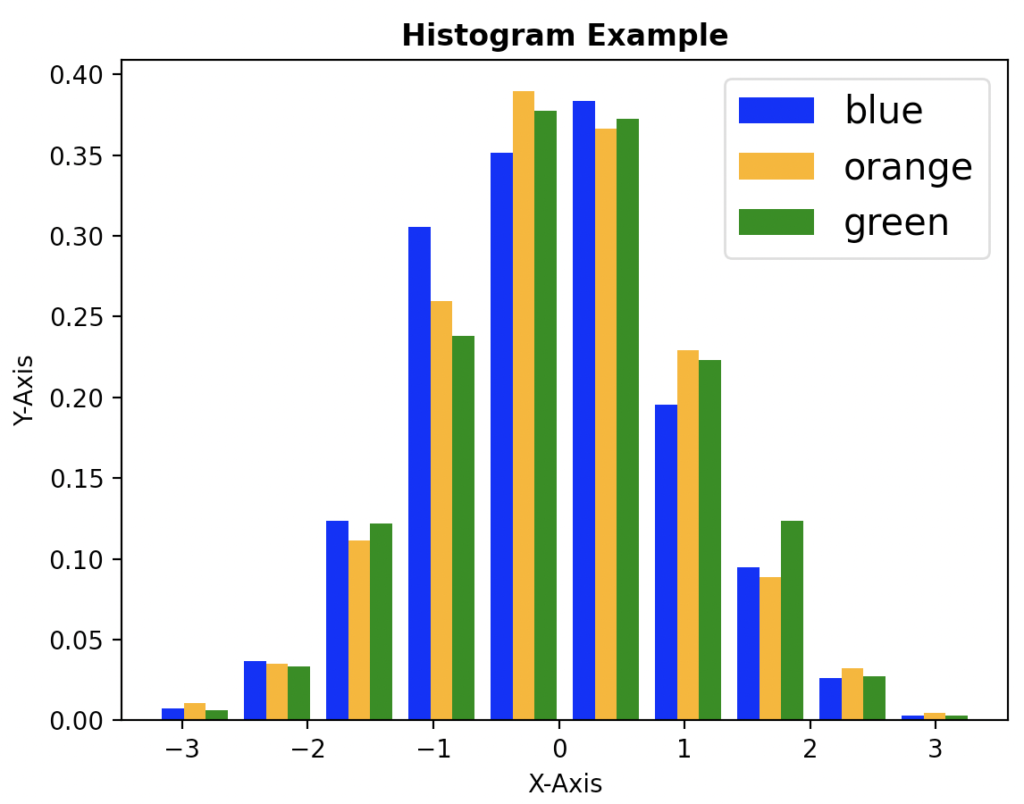# Matplotlib legend: The Complete Guide

0
3A legend in Python Matplotlib is an area describing the elements of the graph. The legend() in Matplotlib is a function that is used to place a legend on the axes.

## Matplotlib legend

Matplotlib.pyplot.legend() is a built-in library function that adds the legend to the graph. The Legend is a description that describes what is used in the graph. The Legend is used to denote the values used on the x-axis and y-axis, which can also describe different colors in the graph.

### Syntax

``matplotlib.pyplot.legend(*args, **kwargs)``

### Arguments

The matplotlib.pyplot.legend() function has no required argument as a parameter. Instead, the legend() function has some optional arguments, some of which are:

1. handles: This is the optional argument. This argument takes a sequence of strings as values. This is used along with the labels argument to plot the legends along with the labels.
2. labels: This is the optional argument. This argument takes a series of strings as values. This argument defines the labels to be used in the legends for the handles.
3. loc: This is the optional argument. This argument takes a string as a value. This argument describes the location in which the Legend is kept.
4. ncols: It takes an integer number as a value. This argument represents the number of columns to be kept in the Legend.
5. labelcolor: This takes a string or list of strings as value. The label colors are passed in this argument.
6. shadow: This takes a Boolean value as a value to this argument. If the value is assigned as True, then a shadow is drawn behind the Legend.
7. title: This is an optional argument. This argument takes string values. The value given in this argument is kept as the title for Legend.
8. fontsize: If the value is an integer, then the fontsize is kept as the value passed in this argument. The fontsize for the Legend is given in this argument.
9. labelcolor: The color for the legend text is given in this argument.
10. facecolor: The legend’s background color is given in this argument. The color given in this argument is kept as the background color for the Legend.

### Return value

The matplotlib.lengend() function returns the legend for the graph.

## Program for creating the legend using matplotlib.pyplot.legend

``````# Importing matplotlib.pyplot as plt.
import matplotlib.pyplot as plt

# Importing numpy as np
import numpy as np

# creating the first line
A = np.array([0, 5, 10, 15, 20])

# creating the second line
B = np.array([0, 1, 3, 4, 8, 12, 16, 20])

# Ploting the line a
plt.plot(A)

# Ploting the line b
plt.plot(B)

# Ploting the legend
plt.legend(["blue", "orange"], loc="center right")

# displaying the created graph using the show method
plt.show()``````

#### OutputIn this program, we imported matplotlib.pyplot for plotting two lines. The matplotlib library consists of all the functions for plotting different types of graphs.

Then, we imported numpy to create two arrays for plotting the lines. Then we plotted the lines using the plot function. Finally, the plot function plots the points into the graph.

Then we plotted the b array. Then we placed the legend with labels blue and orange on the graph. We have placed the legend in the center-right position. Then we have displayed the graph using the show function.

## Program for creating a histogram and then plotting the legends for the histogram using matplotlib.pyplot.legend

``````# Importing matplotlib.pyplot as plt.
import matplotlib.pyplot as plt

# Importing numpy as np
import numpy as np

# Random function is Seeded
np.random.seed(10**7)

# random numbers are created and stored in x variable
x = np.random.randn(1000, 3)

# Number of bins are assigned as 10
num_bins = 10
colr = ["blue", "orange", "green"]

# hist function is used
n, bins, patches = plt.hist(x, num_bins, density=True, color=colr)

# x axis is labeled as X-Axis
plt.xlabel('X-Axis')

# y axis is labeled as Y-Axis
plt.ylabel('Y-Axis')

# Title is kept for the histogram
plt.title('Histogram Example', fontweight="bold")

# Ploting the legend
plt.legend(colr, prop={'size': 15})

# displaying the created graph using the show method
plt.show()``````

#### OutputIn this program, we imported matplotlib.pyplot for plotting the histogram and adding a legend to it.

The matplotlib library consists of all the functions for plotting different types of graphs. Then we have imported numpy for creating random coordinates. Then we created a random array and stored it in x.

Then we have assigned the number of bins as 10. Then we have called the hist function. Then we created a list that is stored in three colors called blue orange and green. This list is stored as a colr array.

The x array is passed as the required argument then we have passed a number of bins as 10 then we have passed density as True then we have passed the color as colr array.

Then we called the legend() function for setting the legend. We also passed the property with a size of 15. In this code, the hist function creates the histogram and the legend function adds the legend to the histogram.

This site uses Akismet to reduce spam. Learn how your comment data is processed.